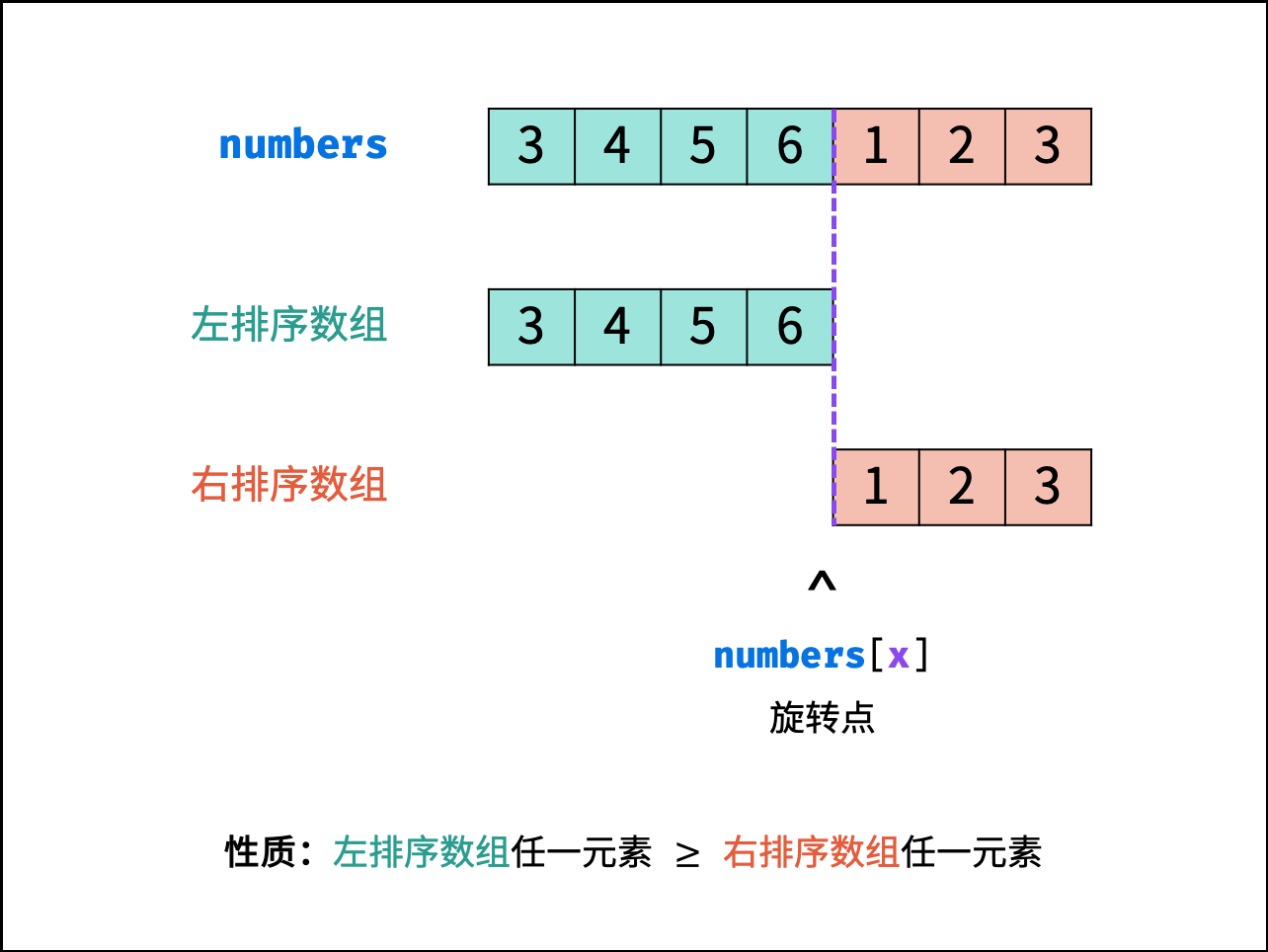0%

# 剑指 Offer 11. 旋转数组的最小数字

## 思路1. 初始化： 声明 i, j 双指针分别指向 nums 数组左右两端；
2. 循环二分： 设 m = (i + j) / 2为每次二分的中点（ "/" 代表向下取整除法，因此恒有 $$i \leq m < j$$ ），可分为以下三种情况：
1. 当 nums[m] > nums[j] 时： m 一定在 左排序数组 中，即旋转点 x 一定在 [m + 1, j] 闭区间内，因此执行 i = m + 1;
2. 当 nums[m] < nums[j]时： m 一定在 右排序数组 中，即旋转点 x 一定在[i, m] 闭区间内，因此执行 j = m；
3. 当 nums[m] = nums[j]时： 无法判断 m 在哪个排序数组中，即无法判断旋转点 x 在 [i, m] 还是 [m + 1, j] 区间中。解决方案： 执行 j -- 缩小判断范围，分析见下文。
3. 返回值： 当 i = j时跳出二分循环，并返回 旋转点的值 nums[i] 即可。

1. 当 x < j 时： 易得执行 j = j - 1 后，旋转点 xx 仍在区间 [i, j]内。

2. 当 x = j 时： 执行 j = j - 1后越过（丢失）了旋转点 x ，但最终返回的元素值 nums[i] 仍等于旋转点值 nums[x] 。

1. 由于 x = j ，因此 $$nums[x] = nums[j] = nums[m] \leq number[i]$$;
2. 又由于 $$i \leq m <j$$恒成立，因此有 m < x ，即此时 m 一定在左排序数组中，因此 $$nums[m] \geq nums[i]$$ ; 综合 1. , 2. ，可推出 nums[i] = nums[m]nums[i]=nums[m] ，且区间 [i, m][i,m] 内所有元素值相等，即有： $nums[i] = nums[i+1] = \cdots = nums[m] = nums[x]$
• 此时，执行 j = j - 1 后虽然丢失了旋转点 xx ，但之后区间 [i, j] 只包含左排序数组，二分下去返回的一定是本轮的 nums[i]，而其与 nums[x] 相等。
• 综上所述，此方法可以保证返回值 nums[i] 等于旋转点值 nums[x] ，但在少数特例下 $$i \ne x$$ ；而本题目只要求返回 “旋转点的值” ，因此本方法正确。